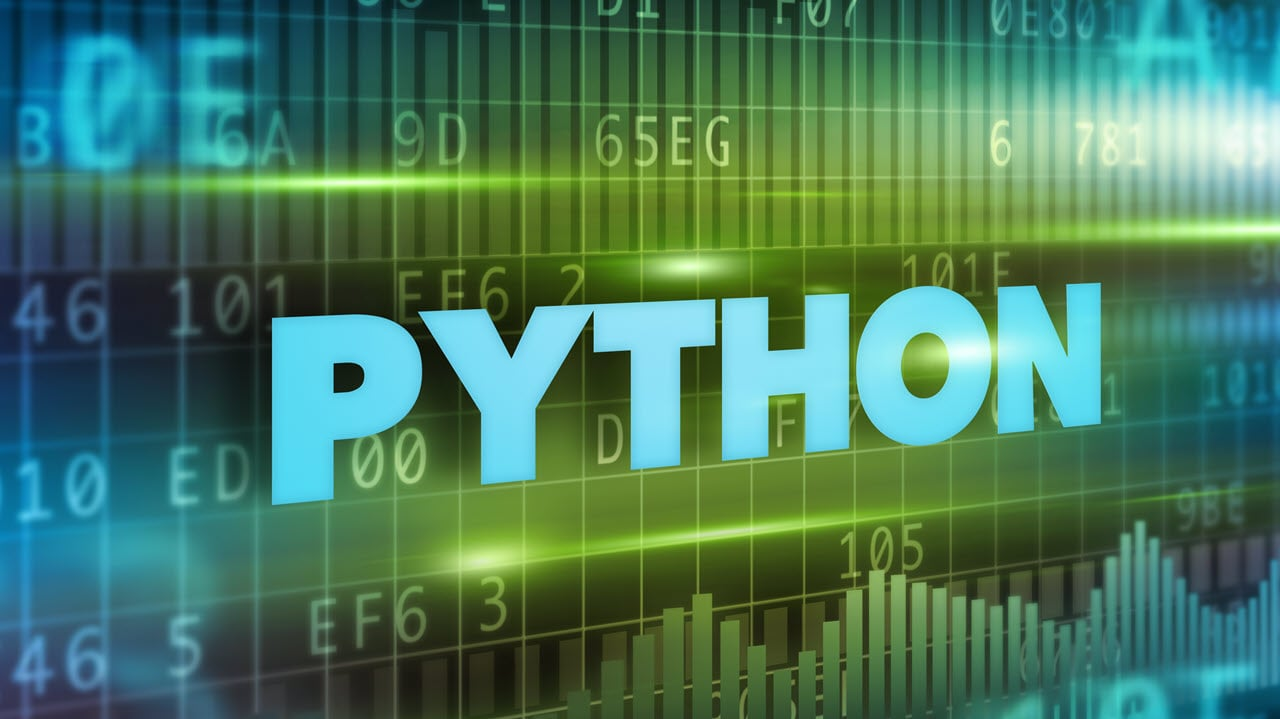# How to convert list to string in Python?Lists and strings are two crucial data types in Python. You often might need to convert one to the other and vice versa. Python, being one of the easiest programming languages around, makes it rather easy for the programmer.

In this article, we’re going over how you can convert a list to a string in Python. There are four ways you can do this.

Also read: How to split Python strings?

# Iterating through the list to convert list to string in Python

This method is the simplest of all and does exactly what it sounds like. You iterate through the list, and on each iteration, extract an element of the list and add it to a string.

``````def listToStringViaIteration(s):
# Making an empty string to store the final result
str1 = ""

# Looping over the list and adding elements to string
for ele in s:
str1 += ele
return str1

# Driver code
s = ['Candid', '.', 'Technology']
print(listToStringViaIteration(s))``````

# Using the join() method to convert list to string in Python

The join() method is a string function that returns a string in which elements of a given sequence have been joined together by the str separator.

``````def listToStringUsingJoin(s):

# Making an empty string to store the final result
str1 = " "
# Using join()
return (str1.join(s))

s = ['Candid', '.', 'Technology']
print(listToStringUsingJoin(s))``````

# Using list comprehension to convert list to string in Python

The aforementioned methods will work fine for as long as your list only contains characters and not integers. If a list does have integers in it, you’re going to have to convert them to a string first before concatenating the entire string together.

The following two methods — list comprehension and the map() function, can tackle this problem.

``````list = ['There', 'are, 7, 'wonders', 'in', 'the', 'world']

# Using list comprehension
listToStrUsingComp = ' '.join([str(elem) for elem in s])

print(listToStrUsingComp)``````

Also read: How to check Python version?

# Using the map() function to convert list to string in Python

The method is quite similar to the join() function; however, it implements a nested map() function, which aids in converting any integers in the list to strings on each given iteration.

``````list = ['There', 'are, 7, 'wonders', 'in', 'the', 'world']

# Using map() function
listToStrUsingMap = ' '.join(map(str, s))

print(listToStrUsingMap)``````

Also read: How to plot multiple lines in Matlab?